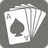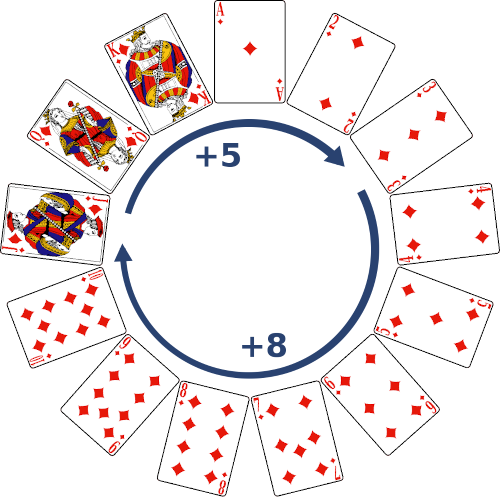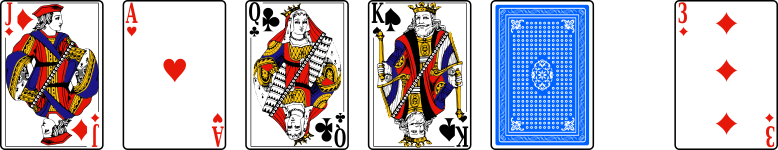Magic with 5 cards
Moderate
English
Magician: John, I'm going to blow your mind with a simple magic trick. Choose five cards.
The magician turns around to see nothing.
John: I just picked five cards.
Magician: Bot will tell me four of them and I will guess the fifth one.
Bot: Jack of diamonds, Ace of hearts, Queen of clubs, King of spades.
Magician: I see red, yeah it's of diamonds... And it's not a face... It's even pretty low... The three of diamonds, right?!
The magician quickly turns around to see John's face...
John: No way!
Magician: Told you!
John: It was just luck, do it again!
Magician: I can do it all day long, dummy.

Well, it's an automatic magic trick, that I will explain in a moment. You will have to create two functions. A function named ``` bot ``` which will take five cards, an integer and return four of them ; and a function named ``` magician ``` which will take four cards, the same integer and return the fifth one.

The trick use the standard 52-card deck . Cards will be represented like ``` 'A ♥' ``` , ``` '3 ♦' ``` , ``` 'K ♠' ``` , ``` 'Q ♣' ``` , ``` 'J ♦' ``` ( ``` ♣ ``` for clubs, ``` ♦ ``` for diamonds, ``` ♥ ``` for hearts, ``` ♠ ``` for spades, A for Ace, J for Jack, Q for Queen and K for King).
We will soon need to compare cards. The deck order is ``` A ♣ < A ♦ < A ♥ < A ♠ < 2 ♣ < ... ``` . To compare two cards, look ranks ``` A < 2 < ... < 10 < J < Q < K ``` , then suits ``` ♣ < ♦ < ♥ < ♠ ``` (same order than with letters: ``` club < diamond < heart < spade ``` ).

The card the bot choose to hide and the order of the four cards the bot says is crucial for the magician so he can guess the fifth one. There are five cards, but only four suits so there are at least two cards from the same suit, we name them card A and card B . The bot will hide one and say the other, but which one? Imagine the thirteen cards in a circle, clockwise . If going from card A to card B on this circle is quicker than going from card B to card A , then we will hide card B , say card A , and the distance from card A to card B is noted as delta (it's necessarily a number between one and six). We still have three cards to say, and since there are six ways to tell three cards, we can transmit "delta information". The card A will be the starting point, then by "adding" delta to card A , the magician can "guess" the fifth card.
Sort the three remaining cards, according to the above order, and note them C1 , C2 and C3 . If delta is 5 or 6, put C1 first, if delta is 3 or 4, put C2 first, and C3 otherwise. You still have two cards to say, tell them in order if delta is odd (1, 3, 5), and in reverse order otherwise (2, 4, 6).
At this point, the bot have two things to say: the card A , and a list of three cards. If we repeat this magic trick multiple times and if we always say the card A first, John might notice it since the fifth card and card A have the same suit. So we are not going to always tell card A first. The first time, we will say it first, the second time, say it second, ..., the fifth time, say it first again...Example: John chooses ``` A ♥ ``` , ``` 3 ♦ ``` , ``` K ♠ ``` , ``` Q ♣ ``` and ``` J ♦ ``` .
• ``` 3 ♦ ``` and ``` J ♦ ``` have the same suit, it's quicker to go from ``` J ♦ ``` to ``` 3 ♦ ``` ( delta = 5 ) than otherwise (8). The starting point is ``` J ♦ ``` and we hide ``` 3 ♦ ``` .
• Remains ``` A ♥ ``` , ``` K ♠ ``` and ``` Q ♣ ``` . Sorted, it's ``` A ♥ ``` , ``` Q ♣ ``` and ``` K ♠ ``` . delta = 5 so C1 = ``` A ♥ ``` first, 5 is odd so the other two in order. So ``` A ♥ ``` , ``` Q ♣ ``` and ``` K ♠ ``` .
• Since it's the first time we do the magic trick, then the bot says ``` J ♦ ``` first, then ``` A ♥ ``` , ``` Q ♣ ``` and ``` K ♠ ``` .
• The magician hears ``` J ♦ ``` , ``` A ♥ ``` , ``` Q ♣ ``` and ``` K ♠ ``` .
• It's the first time he does the magic trick so ``` J ♦ ``` is the starting point. He can say it's of diamonds.
• From ``` A ♥ ``` , ``` Q ♣ ``` , ``` K ♠ ``` order, he deduces delta = 5 (since ``` A ♥ ``` < ``` Q ♣ ``` < ``` K ♠ ``` ). So ``` J ♦ + 5 == 3 ♦ ``` . He says it, with some show.bot magician
Input Five strings and an integer Four strings and an integer
Output A list/tuple of four strings A string

```bot('A ♥', '3 ♦', 'K ♠', 'Q ♣', 'J ♦', n=1) == ['J ♦', 'A ♥', 'Q ♣', 'K ♠']
magician('J ♦', 'A ♥', 'Q ♣', 'K ♠', n=1) == '3 ♦'
```

How it is used: To amaze and impress people with your magic skills.